# NCERT Solutions

## Class 10 science

###### light reflection and refractionQ1) Which one of the following materials cannot be used to make a lens?

1. Water
2. Glass
3. Plastic
4. Clay

(d)clay , A lens allows light to pass through it. Since clay does not show such property, it cannot be used to make a lens

Q2)The image formed by a concave mirror is observed to be virtual, erect and larger than the object. Where should be the position of the object?

1. Between the principal focus and the centre of curvature
2. At the centre of curvature
3. Beyond the centre of curvature
4. Between the pole of the mirror and its principal focus.

(d)
Between the pole of the mirror and its principal focus.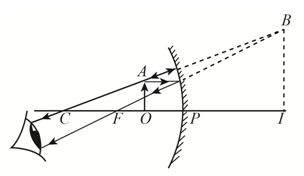Q3)Where should an object be placed in front of a convex lens to get a real image of the size of the object?

1. At the principal focus of the lens
2. At twice the focal length
3. At infinity
4. Between the optical centre of the lens and its principal focus.

(b)
At twice the focal lengthQ4)A spherical mirror and a thin spherical lens have each a focal length of -15 cm. The mirror and the lens are likely to be

1. both concave
2. both convex
3. the mirror is concave and the lens is convex
4. the mirror is convex and the lens is concave.

both concave,: By convention, the focal lengths of a concave mirror and a concave lens are taken as negative. Hence, both, the spherical mirror and the thin spherical lens are concave in nature.

Q5)No matter how far you stand from a mirror, your image appears erect. The mirror is likely to be

1. plane
2. concave
3. convex
4. either plane or convex

either plane or convex,: A convex mirror always gives a virtual and erect image of smaller size than the object placed in front of it. Similarly, a plane mirror always gives a virtual and erect image of same size as that of the object placed in front of it. Therefore, the given mirror could be either plane or convex.

Q6)Which of the following lenses would you prefer to use while reading small letters found in a dictionary?

1. A convex lens of focal length 50 cm
2. A concave lens of focal length 50 cm
3. A convex lens of focal length 5 cm
4. A concave lens of focal length 5 cm

A convex lens of focal length 5 cm. A convex lens gives an erect and magnified image of an object when it is placed between the optical centre and focus of the lens. Also, magnification is more for convex lenses having shorter focal length. Therefore, for reading small letters, a convex lens of focal length 5 cm should be used.

Q7)We wish to obtain an erect image of an object, using a concave mirror of focal length 15 cm. What should be the range of distance of the object from the mirror? What is the nature of the image? Is the image larger or smaller than the object? Draw a ray diagram to show the image formation in this case.

Range of the distance of the object = 0 to 15 cm from the pole of the mirror. Nature of the image = virtual, erect and larger than the object.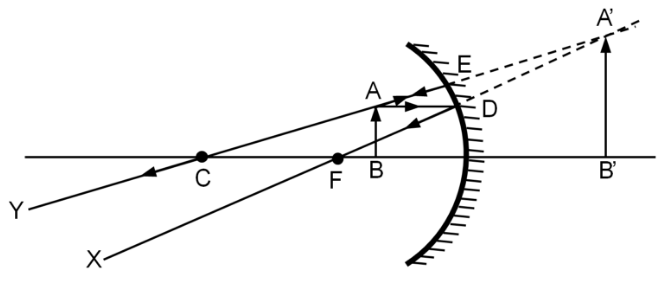In case of concave mirror, the erect & hence the virtual image can be formed only when the object is placed between the principal focus and pole.

Q8)Name the type of mirror used in the following situations.

2. Side/rear-view mirror of a vehicle
3. Solar furnace

(a) Concave
(b) Convex
(c) Concave
Explanation
(a) Concave mirror is used in the headlights of a car. This is because concave mirrors can produce powerful parallel beam of light when the light source is placed at their principal focus.
(b) Convex mirror is used in side/rear view mirror of a vehicle because convex mirrors give a virtual, erect, and diminished image of the objects placed in front of them and have a wide field of view. It enables the driver to see most of the traffic behind him/her.
(c) Concave mirrors are convergent mirrors. That is why they are used to construct solar furnaces. Concave mirrors converge the light incident on them at a single point known as principal focus. Hence, they can be used to produce a large amount of heat at that point.

Q9)One-half of a convex lens is covered with a black paper. Will this lens produce a complete image of the object? Verify your answer experimentally. Explain your observations.

The convex lens will form complete image of an object, even if its one-half is covered with black paper. It can be understood by the following two cases.
Case I :
When the upper half of the lens is covered:
In this case, the rays of light coming from the object will be refracted by the lower half of the lens. These rays meet at the other side of the lens to form the image of the given object, as shown in the following figure.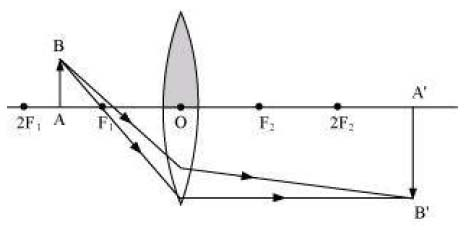Case II
When the lower half of the lens is covered:
In this case, a ray of light coming from the object is refracted by the upper half of the lens. These rays meet at the other side of the lens to form the image of the given object, as shown in the following figure.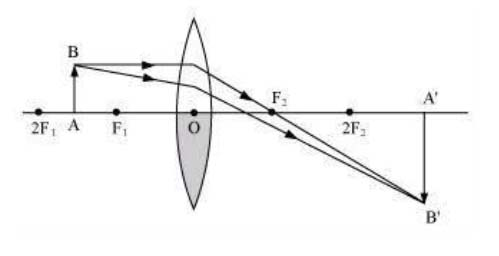In case of the half covered lens, the number of rays used up to make the image on the other side of the lens will be reduced to half.

Q10)An object 5 cm in length is held 25 cm away from a converging lens of focal length 10 cm. Draw the ray diagram and find the position, size and the nature of the image formed.

Object distance, u = -25 cm
Object height, ho = 5 cm
Focal length, f = +10 cm
According to the lens formula,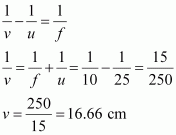The positive value of v shows that the image is formed at the other side of the lens.
Magnification of lens, m=image distance /object distance =v/u
m=v/u=16.66/-25=-0.66
The negative sign shows that the image is real and formed behind the lens.
m = Image height/object height = hi/5;
hi = m x ho = -0.66 x 5 = -3.3 cm
The negative value of image height indicates that the image formed is inverted.
The position, size, and nature of image are shown in the following ray diagram.Q11)A concave lens of focal length 15 cm forms an image 10 cm from the lens. How far is the object placed from the lens? Draw the ray diagram.

Focal length (OF1) of the concave lens is f = -15 cm
Image distance, v = -10 cm
According to the lens formula,Q12)An object is placed at a distance of 10 cm from a convex mirror of focal length 15 cm. Find the position and nature of the image.

Focal length of convex mirror, f = +15 cm
Object distance, u = -10 cm
According to the mirror formula,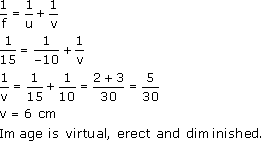The positive value of v indicates that the image is formed behind the mirror. The positive value of magnification indicates that the image formed is virtual and erect. Correct interpretation of the signs are important. Remember: Object distance u is conventionally always taken as negative. Magnification: Negative value corresponds to real & inverted images. Positive value corresponds to virtual & erect images.

Q13)The magnification produced by a plane mirror is +1. What does this mean?

The positive sign means image formed by a plane mirror is virtual and erect. Since the magnification is 1 it means that the size of the image is equal to the size of the object.

Q14)An object 5 cm is placed at a distance of 20 cm in front of a convex mirror of radius of curvature 30 cm. Find the position, nature and size of the image.

Given:
Object distance, u = -20 cm
Object height, H = 5 cm
Radius of curvature, R = 30 cm
Radius of curvature = 2 × Focal length
i.e., R = 2 f
f=R/2=30/2=15cm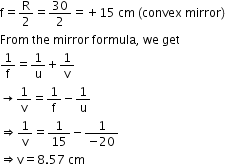The positive value of v indicates that the image is formed behind the mirror.
The positive value of magnification indicates that the image formed is virtual.
m=h'/h
h' = m × h = 0.428 × 5 = 2.14 cm
The positive value of the image height indicates that the image formed is erect.
Therefore, the image formed is virtual, erect and smaller in size.

Q15)An object of size 7.0 cm is placed at 27 cm in front of a concave mirror of focal length 18 cm. At what distance from the mirror should a screen be placed, so that a sharp focused image can be obtained? Find the size and the nature of the image.

Object distance, u= - 27 cm
Object height, h= 7 cm
Focal length, f = - 18 cm
According to the mirror formula,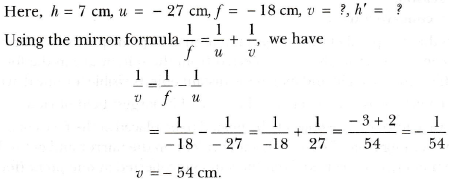The screen should be placed at a distance of 54 cm in front of the given mirror
m = -54/27 = -2
Negative value indicates that image formed is real.
h' = h × m
h' = 7 × (-2) = -14 cm
The negative value of the image height indicates that the image formed is inverted.

Q16)Find the focal length of a lens of power -2.0 D. What type of lens is this?
Power of lens, P = 1/f
P = -2D
f = 1/P
f = -1/2;

f = -0.5 m The focal length is negative. Hence, it is a concave lens. The value of the focal length should be written in metres when substituting in the formula:

Q17)A doctor has prescribed a corrective lens of power +1.5 D. Find the focal length of the lens. Is the prescribed lens diverging or converging ?

Power of lens, P= 1/f
P= 1.5D
f= 1/1.5 = 10/15 = 0.66 m
A convex lens has a positive focal length. Hence, it is a convex lens or a converging lens.